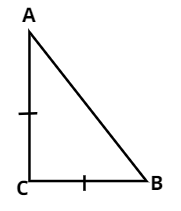Courses
Courses for Kids
Free study material
Free LIVE classes
MoreLIVE
Join Vedantu’s FREE Mastercalss

# $ABC$ is an isosceles triangle with $AC = BC$. If $A{B^2} = 2A{C^2}$, prove that $ABC$ is a right angled triangle.Verified
363.3k+ views
Hint – Use Pythagoras theorem and make a diagram .Given, $\Delta ABC$ is an isosceles triangle .
Also given $AC = BC....(1)$
Also, given that
${(AB)^2} = 2{(AC)^2}$
Using Pythagora's theorem, where square of hypotenuse is equal to sum of the square of other two sides of the right angled triangle.
$\therefore \;{(AB)^2} = {(AC)^2} + {(AC)^2}.....(2)$
Therefore,
From (1) and (2),
${(AB)^2} = {(AC)^2} + {(BC)^2}$
Hence , By converse of Pythagoras theorem triangle $ABC$ is an isosceles right angled triangle.

Note - In these types of questions of isosceles triangles are those whose two sides are equal .Right angled triangle can also be isosceles . Here we have proved the questions by converse of Pythagoras theorem.
Last updated date: 16th Sep 2023
Total views: 363.3k
Views today: 10.63k